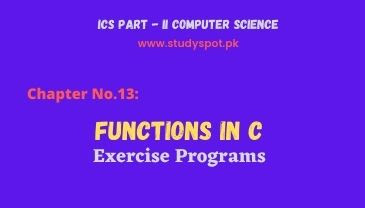# Functions in C - Exercise ProgramsFunctions in C Short Questions with Answers. This post for the students of Computer ICS Part 2 | Computer Science 2nd Year Chapter No.13 Functions in C. Find the exercise solution of Chapter 13: Functions in C, the solution is in Turbo C language for the final examination of intermediate for all boards of Punjab as well as Pakistan.

We tried our best to provide the exercise programs in simple and easy concepts. This post covers the topics i.e., user-defined functions Turbo C such as Is_Prime, Add(), Subtract(), Multiply(), Divide(), Reverse(), Factorial, Draw_Asterisks(), and GCD().

## Chapter No.13: Functions in C

### Exercise Programs | Solution

Q.10: Write a program that prompts the user to enter a number and then reverse it. Write a function Reverse to reverse the number. For example, if the user enters 2765, the function should reverse it so that it becomes 5672. The function should accept the number as an input parameter and return the reversed number.

#include <stdio.h>

#include <conio.h>

int Reverse(int n);

void main(void)

{

int m;

printf("Enter an integer number:");

scanf("%d", &m);

printf("\n\t===================\n");

printf("\n\tReversed Number = %d\n", Reverse(m) );

}

int Reverse(int n)

{

int rev = 0, remainder;

while (n != 0)

{

remainder = n % 10;

rev = rev * 10 + remainder;

n /= 10;

}

return rev;

}

Q.11: Write a function named Draw_Asterisks that will print asterisks (*) according to the pattern shown in the following and make a function call from the function main to print the asterisks pattern.

*********

*******

*****

***

*

#include <stdio.h>

#include <conio.h>

void Draw_Asterisks(void);

void main(void)

{

Draw_Asterisks();

}

void Draw_Asterisks(void)

{

int outer, inner;

for(outer=9; outer>=1; outer-=2)

{

inner=1;

while(inner<=outer)

{

printf("*");

inner++;

}

printf("\n");

}

}

Q.12: Write a function Is_Prime that has an input parameter i.e. num, and returns a value of 1 if num is prime, otherwise returns a value of 0.

#include <stdio.h>

#include <conio.h>

int Is_Prime(int n);

void main(void)

{

int m;

printf("Enter any number:");

scanf("%d",&m);

printf("\n %d",Is_Prime(m));

}

int Is_Prime(int n)

{

int i;

for(i=2;i<n;i++)

{

if(n%i==0)

return 0;

}

return 1;

}

Q.13: Write a complete C program that inputs two integers and then prompts the user to enter his/her choice. If the user enters 1 the numbers are added, for the choice of 2 the numbers are divided, for the choice of 3 the numbers are multiplied, for the choice of 4 the numbers are divided (divide the larger number by the smaller number, if the denominator is zero display an error message), and for the choice of 5, the program should exit. Write four functions Add (), Subtract (), Multiply (); and Divide () to complete the task.

#include <stdio.h>

#include <conio.h>

int Subtract(int m, int n);

int Multiply(int m, int n);

int Divide(int m, int n);

void main(void)

{

int a,b,choice;

printf("Enter Two Numbers:");

scanf("%d %d",&a,&b);

printf("\nEnter 1:Add, 2:Subtract, 3:Multiply, 4:Divide :");

scanf("%d",&choice);

if(choice==1)

else if(choice==2)

printf("\n Subtraction:%d \n",Subtract(a,b));

else if(choice==3)

printf("\n Multiplication:%d \n",Multiply(a,b));

else if(choice==4)

printf("\n Division:%d \n",Divide(a,b));

else

printf("Wrong choice! Plese enter right choice..");

}

{

return (m+n);

}

int Subtract(int m, int n)

{

return (m-n);

}

int Multiply(int m, int n)

{

return (m*n);

}

int Divide(int m, int n)

{

if(m>n)

return (m/n);

else

return (n/m);

}

Q.14: Write a program that prompts the user to enter a number and calls a function Factorial() to compute its factorial. Write the function Factorial () that has one input parameter and returns the factorial of the number passed to it.

#include <stdio.h>

#include <conio.h>

int Factorial(int n);

void main(void)

{

int a;

printf("Enter any integer:");

scanf("%d",&a);

printf("\nFactorial of %d is : %d",a,Factorial(a));

}

int Factorial(int n)

{

int i,fact=1;

for(i=1;i<=n;i++)

{

fact=fact*i;

}

return fact;

}

Q.15: Write a function GCD that has two input parameters and returns the greatest common divisor of the two numbers passed to it. Write a complete C program that inputs two numbers and call the function GCD to compute the greatest common divisor of the numbers entered.

#include <stdio.h>

#include <conio.h>

int GCD(int n1,int n2);

void main(void)

{

int a, b;

printf("Enter two integers: ");

scanf("%d %d", &a, &b);

printf("G.C.D of %d and %d is: %d", a, b, GCD(a,b));

}

int GCD(int n1,int n2)

{

int i,gcd;

for(i=1; i <= n1 && i <= n2; ++i)

{

if(n1%i==0 && n2%i==0)

gcd = i;

}

return gcd;

}